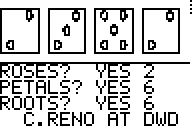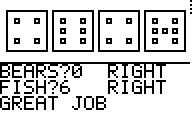BACK

Madison Farrish 9/22/06  chemsitry p. 6

[4/4]

Answer the following fully and completely using nouns, verbs, and ly-words.

[9/10]

1. Complete the table using your Periodic APP or the 50th Year of ChemEd table.
Theses answers came from 50th year ChemEd.

 Element Density Neutrons You Pick Pa [5/5]15.37 g/mL 140[5/5] 4500 K=boiling point[5/5] W [5/5]19.25 g/mL 110[5/5] 6173 K= boiling point[5/5] Fe [5/5]7.87 g/mL 30[5/5] 3136 K= boiling point[5/5] Tm[0/10] 7.265 g/cm3 69 1.96 = Electronegativity

2. Determine the density of the block that Mr. Young assigns you.

V= 7.6*12.7*2=193.04 cm^3

M= 144 g

144/193.04= .74 g/cm^3

I had the Blank Index Cards, and the density of them is .74 g/cm^3. I got the density by dividing the mass—144g--- by the volume---193.04cm^3.

[14/15]

3. If the Lead Brick was really Gold – How heavy would it be? Give the answer in 4 different units.

11.34 g/cm^3 is the density of lead.

The density of gold is 19.3.

19.3* 395.92^3= 1197787100 gà 2.640669E6 lbmà1.197787E6 kgà 8.207449E4 slug à 1.320334E3 ton

I got these answers by using the unit converter on my calculator.

[5/25]

[wrong brick]

4. We are interested in the amount of lead in 55 cans of paint. There should be 0.047 centigrams of lead in each can. Each can has a mass of 0.023 slugs. If we perform a T-Test on the data collected from these cans, at what Level of Significance would you test? Why?

I think it would have a level of significance .04, because the amount of lead in the paint is very important, because lead can be very deadly or toxic. For example, if I painted my fence and my dog came up and licked it a lot, it would die if the lead in the paint wasn’t equal to the amount it needed to be. That’s how toxic lead is.

[15/15]

5. Determine the ppb of lead for the paint in question 4.

First you convert .047 centigrams into grams and .023 slugs into grams. So now I have .00047 grams from my centigrams, and 3.356598E2 g from my slugs. So know you dive .00047g by 335.6598 and times it by a billion.

1.400227254E-6 * 1000000000= 1400.23. The ppb for lead in question 4 is 1400.23

[15/15]

6. Convert these and fill the table

I used the unit converter in my calculator to figure this out.

 To Convert Standard Notation Scientific Notation 0.000555 N [4/5].000124769 pounds [5/5]1.24769E-4 pounds 5.0567E3 g/cm3 [5/5]5056700kg/m3 [5/5]5.0567E6 kg/m3 444.0005 °K [5/5]339.5309°F [5/5]3.395309E2 °F

7. ROSES and BEARS: Use screen shots of each to explain the relationship between Roses/Petals/Roots and Bears/Fish. [Just compare one pair.]I think both roses and fish are alike. To find roses, you look at the middle of the dice to see if there is a middle dot, and if there is one then that dice is a rose. You also look to the middle of the dice when looking for fish. If there is a dot then you look to the right and left of the dot and count how many dots aren’t there.

[12/20]

8. Using the LEAD list, perform a T-Test using the LOS from question 4. Report the results and your conclusion(s).

- After I did the T-test I compared the answer I got p= .0278 to the level of significance I chose, .04. This means that I would accept the amount of lead in each paint-can, because .0278 is lower than the level of significance I chose. [20/20] sweet!]